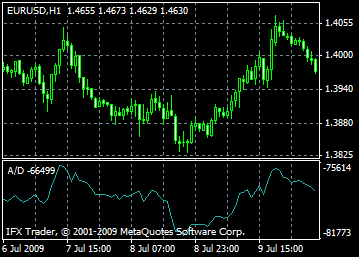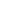# Accumulation/Distribution (A/D)

Accumulation/Distribution (A/D) is a technical indicator determined by a change in price and volume. It should be noted that a weighty factor in the change of value is the volume. Greater ratio (volume) indicates that the impact of price changes on the indicator’s value (over a given period) is quite substantial.

This indicator is rather similar to the famous On Balance Volume. They both are used to confirm price correction by measuring a specific trade volume.

If the Accumulation/Distribution shows growth, it means accumulation or, in other words, a purchase of a stock. The fact is that the lion's share of trading volume is connected with an uptrend. A decline in the indicator shows distribution or a sale of a stock. When the indicator drops, it means distribution (selling) of a security, since most of the volume is associated with downward price movements.

The difference in Accumulation/Distribution values and a stock price signals the soon price correction. As a rule, in case of discrepancy, the price is changed in line with indicator’s direction. For example, if the indicator rises and the stock is falling, you are likely to see a price reversal soon.## Calculation:

To/from the current indicator reading is added/deducted a certain part of day volume. If the closing price is near to a daily high, then the size of added part is larger. If the closing price is near to a daily low – the deducted part is bigger. If the closing price is exactly in between the high and low, the indicator remains at the same level.

A/D(i) =((CLOSE(i) - LOW(i)) - (HIGH(i) - CLOSE(i)) * VOLUME(i) / (HIGH(i) - LOW(i)) + A/D(i-1)

meaning:

A/D(i) - Accumulation/Distribution indicator for the current bar;

CLOSE(i) – bar closing price;

LOW(i) – bar high;

HIGH(i) – bar low;

VOLUME(i) – volume;

A/D(i-1) - Accumulation/Distribution indicator for the previous bar.

All MT5 content